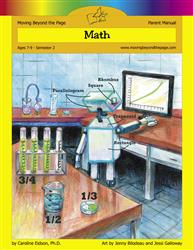# Common Core Alignment

CCSS.Math.Content.3.MD.7.c - Use tiling to show in a concrete case that the area of a rectangle with whole-number side lengths a and b + c is the sum of a × b and a × c. Use area models to represent the distributive property in mathematical reasoning.

## 5: MathUnit 5: Area and Perimeter
Lesson 7: Calculating Area